In this post, I’ll implement feature matching, a simple tehnique to mitigate GANs mode collapse.

### What is mode collapse

Mode collapse is one of the well-known problems in training GANs. In the real world, data distribution may be multi modal (having multiple peaks). In that case, the generator can fool the discriminator by just creating single plausible sample from one of the peaks. As such, when mode collapse happens, the generator’s output tends to be one or some small numbers of similar data.

While I was playing with DCGAN, I experienced this. This is the sample (real) images from OpenAI gym CarRacing environment.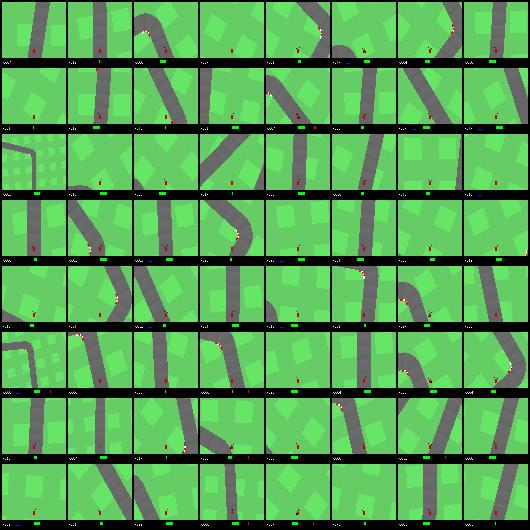And this is the generated images. After at some point of training, the same image is generated again and again.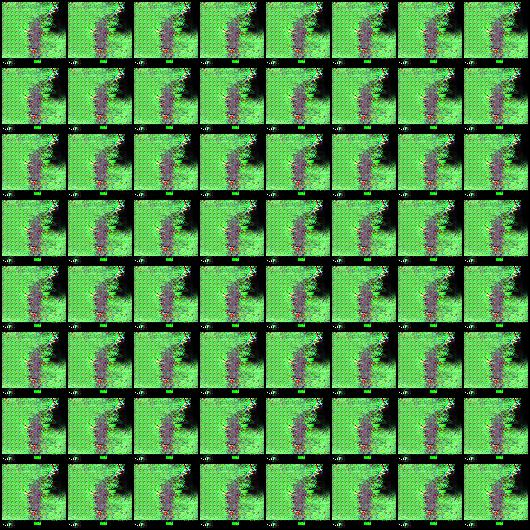The graph below is losses through 300 epochs. This indicates that the generator doesn’t generate a realistic data well.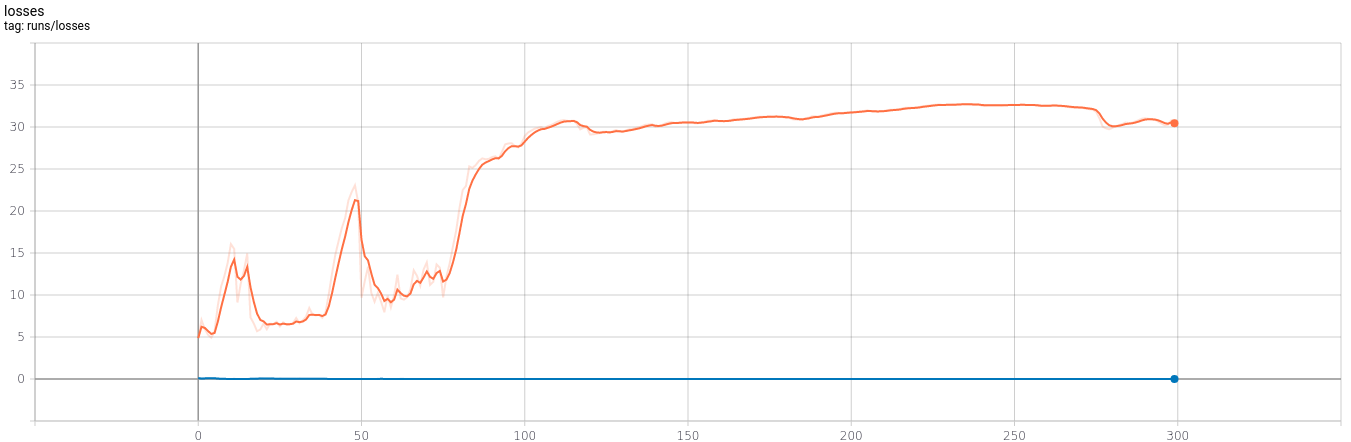### Proposed solutions/mitigations

Some mitigations were suggested from previous studies. Here is the list of them.

• Feature Matching
• Minibatch Discrimination
• WGAN
• Unrolled GAN

In this post, I’ll explore feature matching  a bit (and explore rest of them further in the future posts).

The technique was proposed in the paper “Improved Techniques for Training GANs”. This is fairly simple to implement and easy to see if it makes the situation better.

Instead of directly maximizing the output of the discriminator, the new objective requires the generator to generate data that matches the statistics of the real data, where we use the discriminator only to specify the statistics that we think are worth matching.

In this regard, our loss function is changed to this $\left\Vert \mathbb{E}_{x \sim p_{data}} f(X) - \mathbb{E}_{z \sim p_{z}(z)} f(G(z)) \right\Vert^2_2$ where $f(x)$ denote activations on an intermediate layer of the discriminator.

Let’s try this out. To implement this, first, modify discriminator to return intermediate tensor along with final output.

You can find existing implementation of DCGAN like PyTorch tutorial as your starting point, although the following code is not from tutorial but the main point is to return intermediate tensor so you can copy and paste and fix it.

Then, change your loss function of the generator from BCE to MSE and compute the loss like following way.

Basically, that’s it and here is the experimental results.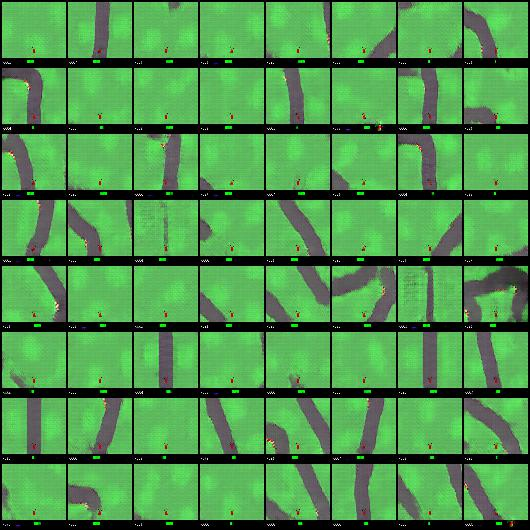Quality of generated images are fine and the losses of both the generator and the discriminator looks good. This simple technique nicely improves the GANs performance.

However, one thing to note is that the loss of the generator started rising at some point and didn’t go down. When I train GANs, I frequently see this kind of situation. It’s one of the problem to determine when to finish GANs training. I’ll study this further.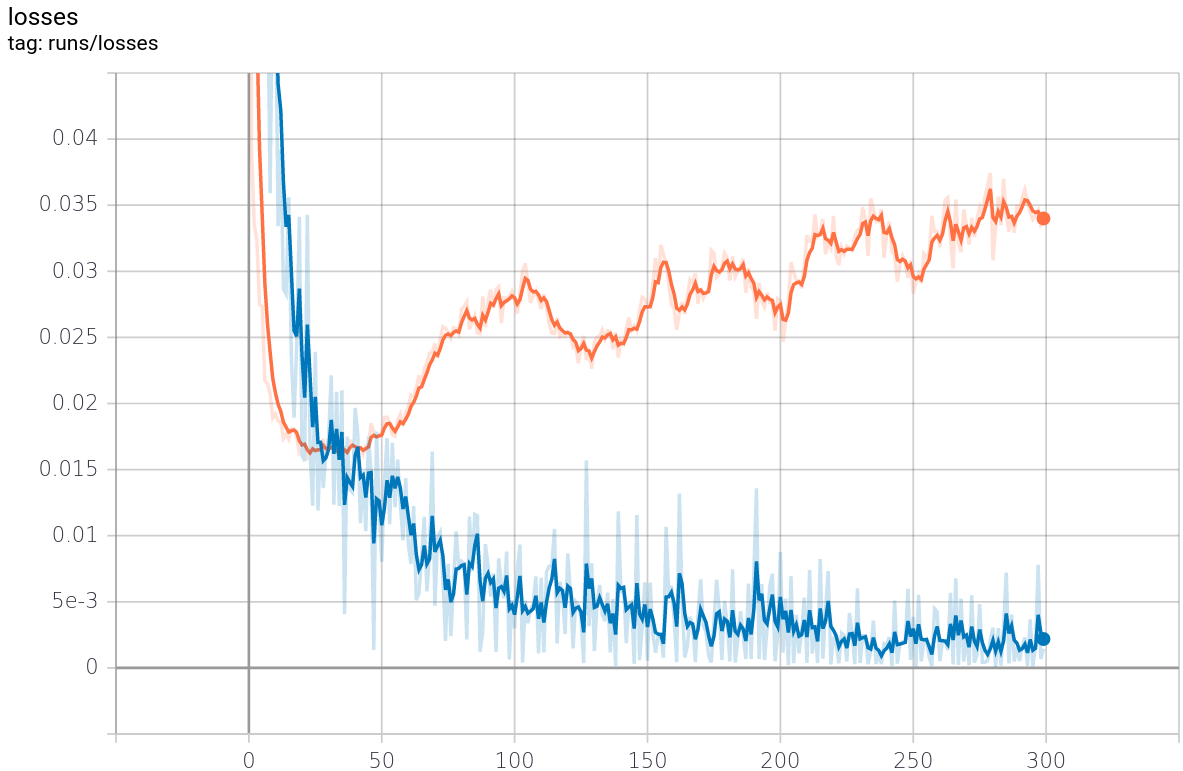In this post, I introduced feature matching and confirmed that this simple approach actually solved mode collapse problem. I’m going to implement other known techniques later.